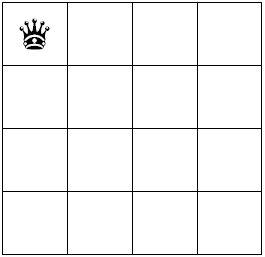# PHP实现八皇后算法

更新时间：2019年05月06日 08:35:41   作者：CyborgLinPHP代码实现：

```<?php

class Backtracking {

protected \$chessboard;  // 棋盘 二维数组 表示坐标轴
protected \$N;      // N表示几皇后
protected \$has_set_x;  // 已经设置的x坐标数组 已经设置的x坐标就不能重复了，用于检查坐标是否可用
protected \$has_set_y;  // 已经设置的y坐标数组 已经设置的y坐标就不能重复了，用于检查坐标是否可用
protected \$has_set_site; // 已经设置的点

function __construct(\$N) {
// 初始化数据
\$this->N = \$N;
\$this->chessboard = array();
for (\$i=0; \$i < \$N; \$i++) {
for (\$j=0; \$j < \$N; \$j++) {
\$this->chessboard[\$i][\$j] = 0;
}
}
\$this->has_set_x = array();
\$this->has_set_y = array();
\$this->has_set_site = array();
}

// 获取排列
public function getPermutation(\$is_get_on = true) { // is_get_on 是否获取一种排列 true:是 false:获取所有排列
\$current_n = 0; // 当前设置第几个皇后
\$start_x = 0;  // 当前的x坐标 从x开始放置尝试
\$permutation_array = array(); // 全部皇后放置成功的排列数组
while (\$current_n < \$this->N && \$current_n >= 0) {
\$site_result = \$this->setQueenSite(\$current_n, \$start_x); // 设置皇后位置
if(\$site_result == true && \$current_n + 1 >= \$this->N) { // 如果最后的皇后位置放置成功则记录信息
\$permutation_array[] = array_merge(\$this->has_set_site, array(array('x' => \$site_result['x'], 'y' => \$site_result['y'])));
if(\$is_get_on == false) { // 如果是获取所有排列，则设置当前放置失败，让程序回溯继续找到其他排列
\$site_result = false;
}
}
if(\$site_result == true) {
\$this->chessboard[\$site_result['x']][\$site_result['y']] = 1;
\$this->has_set_x[] = \$site_result['x'];
\$this->has_set_y[] = \$site_result['y'];
\$this->has_set_site[] = array('x' => \$site_result['x'], 'y' => \$site_result['y']);
\$current_n++; // 皇后位置放置成功，继续设置下一个皇后，重置下一个皇后的x坐标从0开始
\$start_x = 0;
}else {
// 当前皇后找不到放置的位置，则需要回溯到上一步
\$previous_site = array_pop(\$this->has_set_site); // 找到上一步皇后的位置
if(!empty(\$previous_site)) {
\$start_x = \$previous_site['x'] + 1; // 让上一步的皇后的x坐标+1继续尝试放置
\$this->deleteArrayValue(\$this->has_set_x, \$previous_site['x']);
\$this->deleteArrayValue(\$this->has_set_y, \$previous_site['y']);
\$this->chessboard[\$previous_site['x']][\$previous_site['y']] = 0;
}
\$current_n--; // 回溯到上一步，即让一个皇后x坐标+1继续尝试放置
}
}
return \$permutation_array;
}

// 设置皇后位置
public function setQueenSite(\$n, \$start_x) {
\$start_y = \$n;
if(\$start_x >= \$this->N) return false;
\$check_result = \$this->checkQueenSite(\$start_x, \$start_y); // 检查当前是否可放置
if(\$check_result == true) {
return array('x' => \$start_x, 'y' => \$start_y);
}else { // 不可放置，则x坐标+1，继续尝试
\$start_x++;
return \$this->setQueenSite(\$n, \$start_x);
}
}

// 检查皇后位置是否正确
public function checkQueenSite(\$x, \$y) {
// 判断当前坐标的横、纵、斜线是否存在已经放置的皇后
if(in_array(\$x, \$this->has_set_x)) return false;
if(in_array(\$y, \$this->has_set_y)) return false;
\$operate_array = array(
array('operate_x' => '+', 'operate_y' => '+'),
array('operate_x' => '-', 'operate_y' => '-'),
array('operate_x' => '+', 'operate_y' => '-'),
array('operate_x' => '-', 'operate_y' => '+')
);
foreach (\$operate_array as \$key => \$value) {
\$diagonal_x = \$x;
\$diagonal_y = \$y;
while (true) {
eval("\\$diagonal_x=\$diagonal_x {\$value['operate_x']} 1;");
eval("\\$diagonal_y=\$diagonal_y {\$value['operate_y']} 1;");
if(\$diagonal_x >= \$this->N || \$diagonal_y >= \$this->N || \$diagonal_x < 0 || \$diagonal_y < 0) break;
if(\$this->chessboard[\$diagonal_x][\$diagonal_y] == 1) return false;
}
}
return true;
}

// 删除数组元素
public function deleteArrayValue(&\$array, \$value) {
\$delete_key = array_search(\$value, \$array);
array_splice(\$array, \$delete_key, 1);
}

}

\$N = 8; // 8表示获取8皇后的排列组合
\$backtracking = new Backtracking(\$N);
\$permutations = \$backtracking->getPermutation(false);
var_dump(\$permutations); // 输出92种排列```